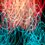# 1, 2, 3, 4, 5 = 2017

Can you use the digits 1, 2, 3 4 and 5 (in some order), along with common mathematical operations, to make 2017?

Allowed:
Concatenation of digits Addition, subtraction, multiplication, division Fractions
Exponents, roots
Factorials

What about just the digits 1, 2, 3 and 4? Is that enough to reach 2017?Note by Chung Kevin
3 years, 10 months ago

This discussion board is a place to discuss our Daily Challenges and the math and science related to those challenges. Explanations are more than just a solution — they should explain the steps and thinking strategies that you used to obtain the solution. Comments should further the discussion of math and science.

When posting on Brilliant:

• Use the emojis to react to an explanation, whether you're congratulating a job well done , or just really confused .
• Ask specific questions about the challenge or the steps in somebody's explanation. Well-posed questions can add a lot to the discussion, but posting "I don't understand!" doesn't help anyone.
• Try to contribute something new to the discussion, whether it is an extension, generalization or other idea related to the challenge.

MarkdownAppears as
*italics* or _italics_ italics
**bold** or __bold__ bold
- bulleted- list
• bulleted
• list
1. numbered2. list
1. numbered
2. list
Note: you must add a full line of space before and after lists for them to show up correctly
paragraph 1paragraph 2

paragraph 1

paragraph 2

[example link](https://brilliant.org)example link
> This is a quote
This is a quote
    # I indented these lines
# 4 spaces, and now they show
# up as a code block.

print "hello world"
# I indented these lines
# 4 spaces, and now they show
# up as a code block.

print "hello world"
MathAppears as
Remember to wrap math in $$ ... $$ or $ ... $ to ensure proper formatting.
2 \times 3 $2 \times 3$
2^{34} $2^{34}$
a_{i-1} $a_{i-1}$
\frac{2}{3} $\frac{2}{3}$
\sqrt{2} $\sqrt{2}$
\sum_{i=1}^3 $\sum_{i=1}^3$
\sin \theta $\sin \theta$
\boxed{123} $\boxed{123}$

Sort by:

$\frac{(4+3)!}{\frac{5}{2}}+1=\boxed{2017}$

The other case seems quite difficult; given that 2017 is a prime number.

- 3 years, 10 months ago

found more by slightly modifying your solution-

$\dfrac{((3!)+2)!}{5 \times 4}+1$,$\dfrac{(3!)\times(4\times2)!}{5!}+1!$

- 3 years, 10 months ago

Very similar to what I did, using 2016+1 :)

- 3 years, 10 months ago

2017, being a prime it is hard!.I've gotten to 2016 so many times like- 2016= $2^5(4^3-1)$

- 3 years, 10 months ago

Wow! Can you add all the different ways that you found?

- 3 years, 10 months ago

hey i got one more for 2017,$(5!+3!)\times 4^{2}+1$

- 3 years, 10 months ago

Works fine :)
Any ideas about the 1,2,3,4 case?

- 3 years, 10 months ago

nope still trying :)

- 3 years, 10 months ago